Normal view

# Computational statistics handbook with MATLAB / Wendy L. Martinez, Angel R. Martinez.

Material type:TextLanguage: English Publisher: Boca Raton, FL : Chapman & Hall/CRC, c2008Edition: 2nd edDescription: xxiii, 767 p. : ill. ; 25 cmISBN: 9781584885665 (alk. paper); 1584885661 (alk. paper)DDC classification: 519.50285 LOC classification: QA276.4 | .M269 2008Online resources: Table of contents only | WorldCat Details | Publisher description | E-book Fulltext
Contents:
Table of contents Introduction -- Probability concepts -- Sampling concepts -- Generating random variables -- Exploratory data analysis -- Finding structure -- Monte Carlo methods for inferential statistics -- Data partitioning -- Probability density estimation -- Supervised learning -- Unsupervised learning -- Parametric models -- Nonparametric models -- Markov chain Monte Carlo methods -- Spatial statistics -- Appendix A : Introduction to MATLAB® -- Appendix B : Projection pursuit indexes -- Appendix C : MATLAB® statistics toolbox -- Appendix D : Computational statistics toolbox -- Appendix E : Exploratory data analysis toolboxes -- Appendix F : Data sets -- Appendix G : Notation.
Summary: Summary: As with the bestselling first edition, "Computational Statistics Handbook with MATLAB" makes computational statistics as accessible as possible by playing down theory and building an understanding of the algorithms used in a wide range of applications. This second edition recognizes the new functionality of the MATLAB[registered] statistics toolbox and other updates like the new plotting capabilities of version 7.0. It includes a discussion of GUI functionality and expanded coverage of topics such as support vector machines, bagging, boosting, and random forests in pattern recognition. The text also features updated and new problem sets, data sets, exercises, and an appendix containing answers to selected problems.
Tags from this library: No tags from this library for this title.Average rating: 0.0 (0 votes)
Item type Current location Collection Call number Copy number Status Date due Barcode Item holdsE-Book
E-book
Non-fiction 519.50285 MAC (Browse shelf) Not For LoanText
Reserve Section
Non-fiction 519.50285 MAC 2008 (Browse shelf) C-1 Not For Loan 25779Text
Reserve Section
Non-fiction 519.50285 MAC 2008 (Browse shelf) C-2 Not For Loan 26048
Total holds: 0
##### Browsing EWU Library shelves, Shelving location: E-book Close shelf browser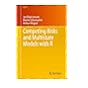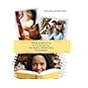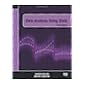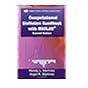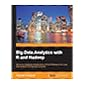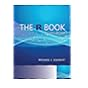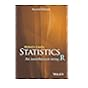519.502 BEC 2012 Competing risks and multistate models with R / 519.50243 AGP 2014 Statistical methods for the social sciences / 519.50285 KOD 2012 Data analysis using stata / 519.50285 MAC Computational statistics handbook with MATLAB / 519.50285 PRB 2013 Big data analytics with R and Hadoop / 519.502855133 CRR 2013 The R book / 519.502855133 CRS 2015 Statistics :

Includes bibliographical references (p. 731-750) and indexes.

Probability concepts --
Sampling concepts --
Generating random variables --
Exploratory data analysis --
Finding structure --
Monte Carlo methods for inferential statistics --
Data partitioning --
Probability density estimation --
Supervised learning --
Unsupervised learning --
Parametric models --
Nonparametric models --
Markov chain Monte Carlo methods --
Spatial statistics --
Appendix A : Introduction to MATLAB® --
Appendix B : Projection pursuit indexes --
Appendix C : MATLAB® statistics toolbox --
Appendix D : Computational statistics toolbox --
Appendix E : Exploratory data analysis toolboxes --
Appendix F : Data sets --
Appendix G : Notation.

Summary:
As with the bestselling first edition, "Computational Statistics Handbook with MATLAB" makes computational statistics as accessible as possible by playing down theory and building an understanding of the algorithms used in a wide range of applications. This second edition recognizes the new functionality of the MATLAB[registered] statistics toolbox and other updates like the new plotting capabilities of version 7.0. It includes a discussion of GUI functionality and expanded coverage of topics such as support vector machines, bagging, boosting, and random forests in pattern recognition. The text also features updated and new problem sets, data sets, exercises, and an appendix containing answers to selected problems.

Applied Statistics

There are no comments for this item.